Pay with
Markets
Derivatives
Earn
NFT
Institutional
Feed

# FAQAccount FunctionsTutorialBinance Fan TokenBinance EarnCrypto Deposit/Withdrawal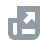Crypto Derivatives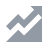FinanceAPISecurityOther Topics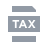Tax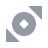Binance Convert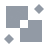NFTVIP
Home
Support Center
FAQ
Binance Margin Level and Margin Call

# Binance Margin Level and Margin Call

2019-07-05 11:08
Margin trading allows you to leverage your positions to amplify market movements. Binance uses Margin Level to evaluate the risk level of your Margin Account.

## 1. Margin Level of Cross Margin

1.1 Margin users can use their Cross Margin account assets as collateral assets for Cross Margin trading. For more details, please refer to the Margin Data page.
1.2 The Margin Level of the Cross Margin Account = Total Asset Value of the Cross Margin Account / (Total Liabilities + Outstanding Interest), where:
Total Asset Value of the Cross Margin Account = Current Total Market Value of All Digital Assets in the Cross Margin Account
Total Liabilities = Current Total Market Value of All Outstanding Margin Loans in the Cross Margin Account
Outstanding Interest = Amount of Each Margin Loan * Loan Time (in hours, at the time of the calculation) * Hourly Interest Rate - Deduction/Paid Interest
Your Margin Level is a key risk metric for your margin account. If it falls below a certain threshold, it will be used to trigger Margin Calls and Liquidations.
1.3 The Collateral Value Ratio will determine the maximum amount you can withdraw from your Cross Margin account.
Collateral Value refers to the total value of all assets in your Cross Margin account in USDT. The Collateral Value also takes into account the relevant Collateral Ratio, the percentage at which the relevant asset is valued. For more details on the Collateral Ratio for each Digital Asset, please refer to the Margin Data page.
The Collateral Value Ratio of the Cross Margin Account = Collateral Value / (Total Liabilities + Outstanding Interest), where:
Collateral Value = Current Total Market Value of All Digital Assets in the Cross Margin Account * Collateral Ratio (calculated separately for each asset and then aggregated)
Total Liabilities = Current Total Market Value of All Outstanding Margin Loans in the Cross Margin Account
Outstanding Interest = Amount of Each Margin Loan * Loan Time (in hours, at the time of the calculation) * Hourly Interest Rate - Interest Paid
1.4 Effect of Margin Level and Collateral Value Ratio
When your Collateral Value Ratio > 2, you can transfer the excess assets to your Spot Wallet, provided the Collateral Value Ratio remains above 2 after the transfer.
When 1.3 < Margin Level ≤ 1.5, you can trade but can’t borrow additional funds.
A margin call may be triggered when 1.1 < Margin Level ≤ 1.3 if you’ve not specified a custom margin call level. And you may be required to transfer in more collateral assets and/or reduce the outstanding loan balance to avoid liquidation. After the first notification, you’ll receive a notification every 24 hours until the Margin Level exceeds 1.3.
When Margin Level ≤ 1.1, liquidation will be triggered, and your collateral assets will be liquidated to pay back the outstanding loan amount and interest. In most cases, the liquidation process will stop when the Margin Level is restored to 1.5 or higher.

## 2. Margin Level of Isolated Margin

2.1 The assets in your Isolated Margin account can only be used as the collateral in the corresponding account. The assets in your other accounts, Cross Margin or other Isolated accounts, can’t be used as collateral.
2.2 The Margin Level of the Isolated Margin Account = Total Value of Assets in the Isolated Margin Account / (Total Liabilities + Unpaid Interest), where:
Total Value of Assets = Current Total Market Value of All Digital Assets in the Isolated Margin Account
Total Liabilities = Current Total Market Value of All Outstanding Margin Loans in the Isolated Margin Account
Outstanding Interest = Amount of Each Margin Loan * Loan Time (in hours, at the time of the calculation) * Hourly Interest Rate - Deduction/Paid Interest
2.3 Margin Level
When the Margin Level (ML) > 2, you can trade, borrow and transfer the excess assets to your Spot Wallet, but only if the ML remains above 2 after the transfer.
When the Margin Call Ratio (MCR) < ML ≤ 2, you can trade and borrow, but can’t transfer assets from the account. The MCR varies for different leverage levels. For example, the MCR for 3x leverage is 1.35. For 5x leverage, it’s 1.18; For 10x leverage, it’s 1.09.
A margin call may be triggered when the Liquidation Ratio (LR) < ML ≤ MCR. You may be required to transfer in more collateral assets and/or reduce the outstanding loan balance to avoid liquidation. After the first notification, you will receive a notification every 24 hours until ML > MCR.
Liquidation will be triggered when ML ≤ LR, and your collateral assets will be liquidated to pay back the outstanding loan amount and interest. Please refer to Tiered Leverage Function on Isolated Margin for more information.